# E-PolyLearning

 51. Choose the figure which is different from the rest.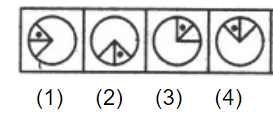a. 1 b. 2 c. 3 d. 4

 52. Choose the figure which is different from the rest.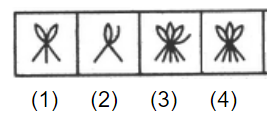a. 1 b. 2 c. 3 d. 4
 53. Choose the figure which is different from the rest.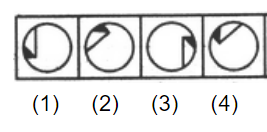a. 1 b. 2 c. 3 d. 4
 54. Choose the figure which is different from the rest.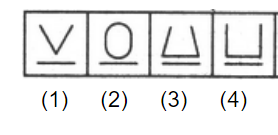a. 1 b. 2 c. 3 d. 4
 55. Choose the figure which is different from the rest.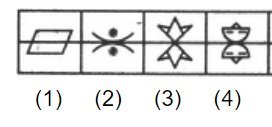a. 1 b. 2 c. 3 d. 4